# Kolmogorov duality

Jump to: navigation, search

A duality in algebraic topology consisting in the isomorphism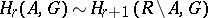between the-dimensional homology group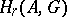of a closed setin a locally compact Hausdorff spacewith zero- and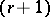-dimensional homology groups, and the-dimensional homology group of the complement, with Abelian coefficient group, as well as the isomorphismbetween the corresponding cohomology groups, with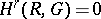and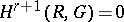.

The homology and cohomology groups involved in these isomorphisms are defined as follows. An-dimensional chain is taken to be any function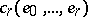of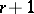subsets of the spacehaving compact closures that is skew-symmetric and additive in each of its arguments, takes values in, and is equal to zero when the intersection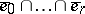is empty. The boundary operator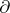is defined by the formula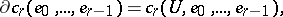whereis any open subset ofwith compact closure containing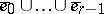. The cycles are the chains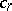with zero boundaries,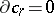, and the cycles homologous to zero are the chainsthat are boundaries,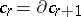. The group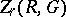of all-dimensional cycles with the usual addition of functions contains the groupof all-dimensional boundaries as a subgroup. The quotient group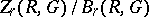is the group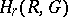. A.N. Kolmogorov always considered the groupto be compact, and compactly topologized the homology group as well. However, the topology of the coefficient group has no effect on the structure of the homology group, and the homology can be taken over any group.

For the definition of cochains one considers the skew-symmetric functionsofpointsof the spacewith values insuch that there exists for each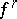a finite systemof pairwise-disjoint subsets ofwith compact closures and satisfying the condition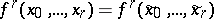ifand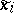belong to the same element of the system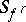for any;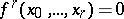if at least one of the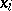is not contained in any element of. The coboundary operatoris defined by the formula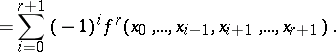Two functions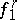and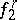are regarded as equivalent if each point inhas a neighbourhoodsuch that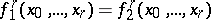whenever all thebelong to, and a cochain is taken to be an equivalence class of functions. The boundary of a cochain is defined as the class of coboundaries entering into this cochain of functions. A cocycle is a cochainwith zero coboundary and a cocycle is cohomologous to zero if it is a coboundary,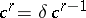. The group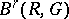of all-dimensional coboundaries is a subgroup of the groupof all-dimensional cocycles; the quotient groupthen defines the group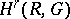.

The homology and cohomology groups defined in this way, which is often referred to as the functional way, were introduced by Kolmogorov . He then went on to prove that, apart from the indicated duality isomorphism, there is a duality between the homology and cohomology groupsandin the sense of the Pontryagin theory of characters, when the compact groupis dual to the group, and the Poincaré duality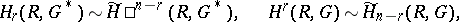whereis an open-dimensional manifold,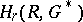andare functional groups over a compact (or discrete) group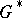(or), and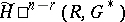and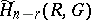are the cohomology (homology) groups of infinite cochains (finite chains) of an arbitrary cellular decomposition of.

In the case whenis the-dimensional Euclidean space, one obtains from the above dualities the Pontryagin duality theorem (see Alexander duality). A special case of these dualities is the Steenrod duality theorem (see Duality in algebraic topology), since the Kolmogorov duality for homology is valid also for arbitrary coefficient groups .

The functional homology groups are isomorphic: to the Vietoris groups (see Vietoris homology) in the case of compact metric spaces and compact coefficient groups ; to the Aleksandrov spectral homology groups with respect to singular subcomplexes  in the case of locally compact spaces and compact coefficient groups  and, consequently, to the Aleksandrov–Čech homology groups of the one-point compactification of a given locally compact space ; and to the Steenrod homology groups  in the case of compact metric spaces and arbitrary groups . Thus, the Kolmogorov homology groups introduced four years earlier than those of Steenrod are a generalization of the latter to a wider class of spaces.

The functional homology and cohomology groups satisfy all the Steenrod–Eilenberg axioms on the category of locally compact spaces with admissible mappings (that is, when the pre-image of each compact set is compact)  and in addition the two Milnor axioms on the category of compact metric spaces .